StatLect

# Loss function

In statistics and machine learning, a loss function quantifies the losses generated by the errors that we commit when:

• we estimate the parameters of a statistical model;

• we use a predictive model, such as a linear regression, to predict a variable.

The minimization of the expected loss, called statistical risk, is one of the guiding principles in statistical modelling.## Example: loss functions in linear regression

In order to introduce loss functions, we use the example of a linear regression modelwhereis the dependent variable,is a vector of regressors,is a vector of regression coefficients andis an unobservable error term.

### Estimation losses

Suppose that we use some data to produce an estimateof the unknown vector.

In general, there is a non-zero differencebetween our estimate and the true value, called estimation error.

Of course, we would like estimation errors to be as small as possible. But how do we formalize this preference?

We use a functionthat quantifies the losses incurred because of the estimation error, by mapping couplesto the set of real numbers.

Typically, loss functions are increasing in the absolute value of the estimation error and they have convenient mathematical properties, such as differentiability and convexity.

An example (whenis a scalar) is the quadratic loss### Prediction losses

After we have estimated a linear regression model, we can compare its predictions of the dependent variable to the true values.

Given the regressors, the prediction ofisThe differencebetween the prediction and the true value is called prediction error.

As in the case of estimation errors, we have a preference for small prediction errors. We formalize it by specifying a loss functionthat maps couplesto real numbers.

Most of the functions that are used to quantify prediction losses are also used for estimation losses.

## Risk and empirical risk

The expected value of the loss is called risk.

Whenis seen as an estimator (i.e., a random variable whose realization is equal to the estimate), the expected valueis the risk of the estimator.

The other relevant quantity is the risk of the predictionswhich can be approximated by the empirical risk, its sample counterpart:whereis the sample size.

## Empirical risk minimization

In a linear regression model, the vector of regression coefficients is usually estimated by empirical risk minimization.

The predictionsdepend onand so does the empirical risk. We search for a vectorthat minimizes the empirical risk.

The Ordinary Least Squares (OLS) estimator ofis the empirical risk minimizer when the quadratic loss (details below) is used as the loss function.

In fact, the OLS estimator solves the minimization problem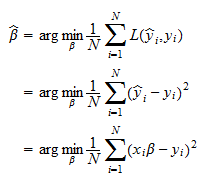Under the conditions stated in the Gauss-Markov theorem, the OLS estimator is also the unbiased estimator that generates the lowest expected estimation losses, provided that the quadratic loss is used to quantify the latter.

## Generalizing the example

What we have said thus far regarding linear regressions applies more in general to:

In other words, given a parametric statistical model, we can always define a loss function that depends on parameter estimates and true parameter values.

Given a predictive model, we can use a loss function to compare predictions to observed values.

We now introduce some common loss function.

We will always use thenotation, but most of the functions we present can be used both in estimation and in prediction.

It is important to note that we can always multiply a loss function by a positive constant and/or add an arbitrary constant to it. These transformations do not change model rankings and the results of empirical risk minimization. In fact, the solution to an optimization problem does not change when the said transformations are performed on the objective function.

The most popular loss function is the quadratic loss (or squared error, or L2 loss).

Whenis a scalar, the quadratic loss isWhenis a vector, it is defined aswheredenotes the Euclidean norm.

When the loss is quadratic, the expected value of the loss (the risk) is called Mean Squared Error (MSE).

The quadratic loss is immensely popular because it often allows us to derive closed-form expressions for the parameters that minimize the empirical risk and for the expected loss. This is exactly what happens in the linear regression model discussed above.

Squaring the prediction errors creates strong incentives to reduce very large errors, possibly at the cost of significantly increasing smaller ones.

For example, according to the quadratic loss function, Configuration 2 below is better than Configuration 1: we accept a large increase in(by 3 units) in order to obtain a small decrease in(by 1 unit).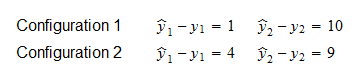This kind of behavior makes the quadratic loss non-robust to outliers.

## Absolute loss

The absolute loss (or absolute error, or L1 loss) is defined aswhenis a scalar and aswhenis a vector.

When the loss is absolute, the expected value of the loss (the risk) is called Mean Absolute Error (MAE).

Unlike the quadratic loss, the absolute loss does not create particular incentives to reduce large errors, as only the average magnitude matters.

For example, according to the absolute loss, we should be indifferent between Configuration 1 and 2 below. An increase in the magnitude of a large error is acceptable if it is compensated by an equal decrease in an already small error.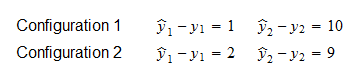The absolute loss has the advantage of being more robust to outliers than the quadratic loss.

However, the absolute loss does not enjoy the same analytical tractability of the quadratic loss.

For instance, when we use the absolute loss in linear regression modelling, and we estimate the regression coefficients by empirical risk minimization, the minimization problem does not have a closed-form solution. This kind of approach is called Least Absolute Deviation (LAD) regression. You can read more details about it on Wikipedia.

## Huber loss

The Huber loss is defined as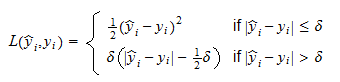whereis a positive real number chosen by the statistician (if the errors are expected to be approximately standard normal, but there are some outliers,is often deemed a good choice).

Thus, the Huber loss blends the quadratic function, which applies to the errors below the threshold, and the absolute function, which applies to the errors above.

In a sense, it tries to put together the best of both worlds (L1 and L2). Indeed, empirical risk minimization with the Huber loss function is optimal from several mathematical point of views in linear regressions contaminated by outliers.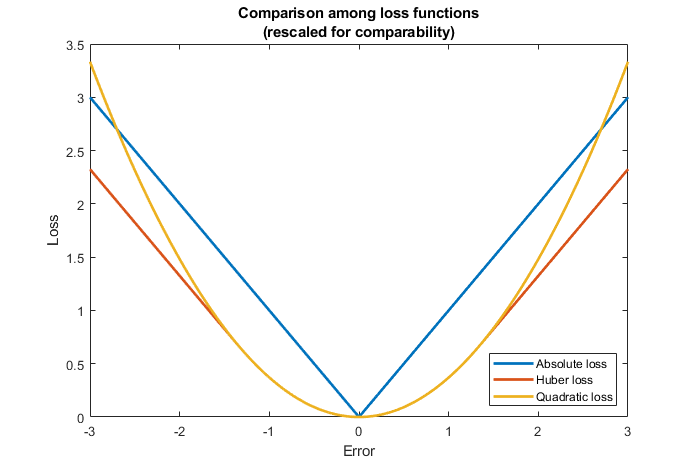## Other loss functions used in regression models

There are several other loss functions commonly used in linear regression problems. For example:

• the log-cosh loss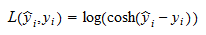which is very similar to the Huber function, but unlike the latter is twice differentiable everywhere;

• the pseudo-Huber loss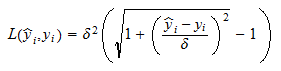which also behaves like the L2 loss near zero and like the L1 loss elsewhere;

• the epsilon-insensitive loss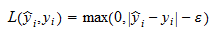whereis a threshold below which errors are ignored (treated as if they were zero); the intuitive idea is that a very small error is as good as no error.

## Loss functions used in classification

Other loss functions are used in classification models, that is, in models in which the dependent variableis categorical (binary or multinomial).

The most important are:

• the log-loss (or cross-entropy)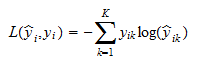whereis amultinoulli vector (when the true category is the-th, thenand all the other entries of the vectorare zero), andis a vector of predictions;

• the hinge loss (or margin loss)which can be used when the variablecan take only two values (or).

## More details

More details about loss functions, estimation errors and statistical risk can be found in the lectures on Point estimation and Predictive models.

Previous entry: Log likelihood

Next entry: Marginal distribution function